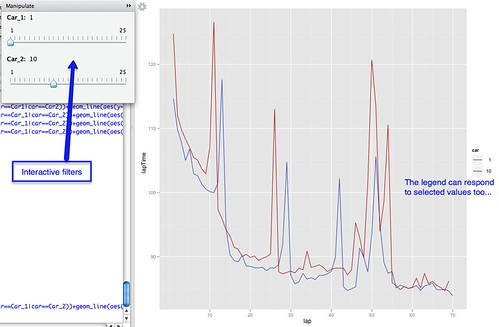Creating Simple Interactive Visualisations in R-Studio: Subsetting Data

Watching a fascinating Google Tech Talk by Hadley Wickham on The Future of Interactive Graphics in R – A Joint Visualization and UseR Meetup, I was reminded of the manipulate command provided in R-Studio that lets you create slider and dropdown widgets that in turn let you dynamically interact with R based visualisations, for example by setting data ranges or subsetting data.

Here are a couple of quick examples, one using the native plot command, the other using ggplot. In each case, I’m generating an interactive visualisation that lets me display as a line chart two user selected data series from a larger data set.Here’s a crude first attempt using plot:

View(hun_2011comprehensiveLapTimes)

library("manipulate")
h=un_2011comprehensiveLapTimes

manipulate(
plot(lapTime~lap,data=subset(h,car==cn1),type='l',col=car) +
lines(lapTime~lap,data=subset(h,car==cn2 ),col=car),
cn1=slider(1,25),cn2=slider(1,25)
)

This has the form manipulate(command1+command2, uiVar=slider(min,max)), so we see for example two R commands to plot the two separate lines, each of them filtered on a value set by the correpsonding slider variable.

Note that we plot the first line using plot, and the second line using lines.

The second approach uses ggplot within the manipulate context:

manipulate(
ggplot(subset(h,h\$car==Car_1|car==Car_2)) +
geom_line(aes(y=lapTime,x=lap,group=car,col=car)) +
Car_1=slider(1,25),Car_2=slider(1,25)
)

In this case, rather than explicitly adding additional line layers, we use the group setting to force the display of lines by group value. The initial ggplot command sets the context, and filters the complete set of timing data down to the timing data associated with at most two cars.

We can add a title to the plot using:

manipulate(
ggplot(subset(h,h\$car==Car_1|car==Car_2)) +
geom_line(aes(y=lapTime,x=lap,group=car,col=car)) +